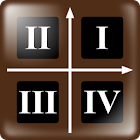Math Word Decode Fun

All Android applications categories

All Android games categories# Math Word Decode Fun

by: 6 6

6 Users
rating

## Screenshots

Description

*** How to Play ***
[Summary]
This game picks most characters of a language, set each of them a number, starts from zero(for example,set alphabet "a" as 0, "b" as 1 ... "_" as 27, " ' " as 28 etc.), and then encode each character of a word(question) by linear equation "y=ax+b", making the original word an unknown encoded word.

[Target]
Decode each character of an encoded word(question), recover the original word from the encoded word.

[Step 1]
Slide the question word, choose a character you want to decode.

[Step 2]
Switch to "table"(character - number cross-references) tab, slide the screen to find out corresponded number of the character you chose at step 1.

[Step 3]
Switch to "Game" tab, substitute the value you found at step 2 into x or y of equation "y=ax+b" and solve for y or x. If the character you chose at step 1 was on line "x", substitute the value into x then solve for y; If the character you chose at step 1 was on line "y", substitute the value into y then solve for x.(ONLY level - Easy(straight) questions are given line "x" to solve for y, others are given line "y" to solve for x.)

[Step 4]
Switch to "table"(character - number cross-references) tab, slide the screen to find out corresponded character by value x or y that you solved at step 3. It is IMPORTANT to know that if value were greater than character count of that language, characters will cycle from the beginning again. For example, in alphabet, value 27 correcpond to "_", 28 correspond to " ' ", 29 correspond to "a", 30 correspond to "b" ... and so on. Additionally, if value were negative, characters will go from end to beginning. For example, in alphabet, value -1 correcpond to " ' ", -2 correcpond to "_" ... -28 correcpond to "b", -29 correcpond to "a", -30 correcpond to " ' ", -31 correcpond to "_" ... and so forth.

[Step 5]
Switch to "Game" tab, slide the character buttons below to select the character you found at step 4, then press the arrow button above to fill this character into question word line.

[Step 6]
Repeat step 1 to step 5 mentioned above until all characters of the question word were filled, and the original word were recovered. Finally press the "Answer" button to judge if your answer were correct or not. You can also fill characters directly without calculation if you have expected what the original word was after solving some characters of the question word.

[P.S.]
The "a" coefficient in linear equation "y=ax+b" is 1 or -1 when game level is "Easy"; is between 9 and -9 (except 0) when game level is "Normal". The "b" coefficient in linear equation "y=ax+b" is between positive and negative of characters count of the language minus 1. For example, in alphabet, "b" is between -28 and 28 (and might be 0); in bopomofo(注音), "b" is between -40 and 40(also might be 0). In game level "Hard", the rule of linear equation "y=ax+b" is the same as level "Normal", but won't show you the "a" and "b" coefficients, just show you "y=ax+b" text. Player have to solve for "a" and "b" with the given "question-answer" character pair, by "linear equations in two variables" solution, get a possible linear equation then substitute value into x or y then solve for y or x.

[Example]
The equation is "y=x+3", and the question word is shown on line x as "vlr". Substitute the "v" corresponded value 21 into x and solve for y as 24, then find out value 24 corresponded character "y"; Substitute the "l" corresponded value 11 into x and solve for y as 14, then find out value 14 corresponded character "o"; Substitute the "r" corresponded value 17 into x and solve for y as 20, then find out value 20 corresponded character "u". Finally you can know the answer is "you".

Tags: zoom math 500 decoder , 文字解碼 , math equation decoding game , 成語解碼;數字 , math words game

from 6 reviews

"Good"

6